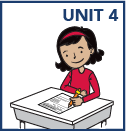top of page

• Use models and strategies to divide 2- and 3-digit numbers by 2-digit numbers, with and without remainders. (Students are not expected to use the standard long division algorithm until sixth grade.)

• Add and subtract fractions with unlike denominators

• Multiply fractions and divide fractions in simple cases

• Measure volume

• Explain patterns in the number of zeros and the placement of the decimal point in the answer when multiplying or dividing by powers of 10

• Read, write, round, compare, add & subtract, multiply & divide decimals

• Multiply multi-digit whole numbers

• Locate and graph points on a coordinate planebottom of page